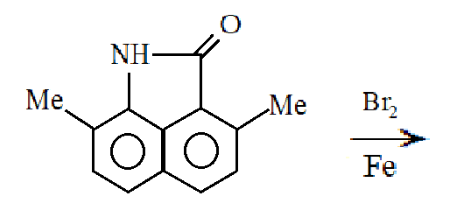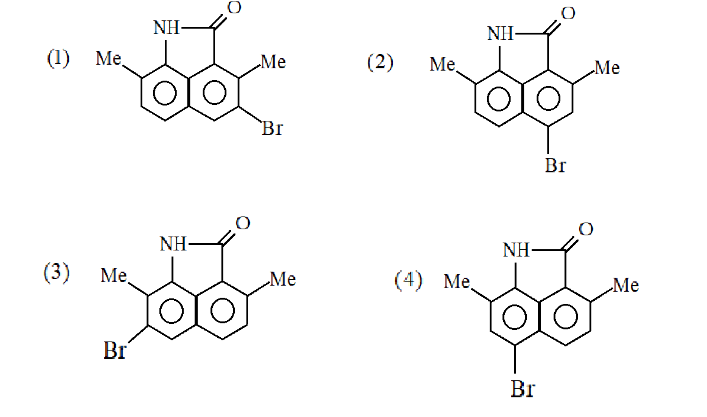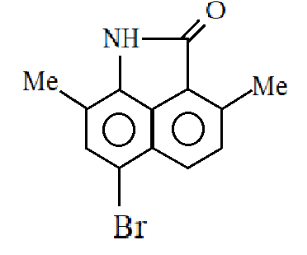QuestionAnswers

# What will be the major product of the given reaction:Verified
91.8k+ views
Hint:We know that this reaction is a bromination reaction for benzene containing compounds. The bromine and iron present will react to generate the $B{r^ + }$ ion. Here $B{r^ + }$ will act as an electrophile so electrophilic substitution will take place in this reaction. To see where the electrophile will get substituted, we need to know about the electron donating group and electron withdrawing group.

Complete step-by-step answer:We already know that $B{r^ + }$ ion has a low electron density and the two benzene rings have a high electron density so the benzene ring will donate its electron to the $B{r^ + }$ ion and hence an electrophile will be substituted on the benzene ring but since the molecule contains two benzene rings we need to see that which benzene will donate its electron to the $B{r^ + }$ ion.
In the first benzene ring, a methyl group is present and a nitrogen is attached with hydrogen. Both the groups are electron donating groups as the lone pairs present on the nitrogen will be delocalized and hence will come in resonance with the benzene ring. Hence both the electron donating group will activate the benzene for the electrophilic substitution for electrophilic substitution reaction.
0n the other hand $C = O$ is present on the second benzene ring and we know that oxygen possesses a high electronegativity so it will pull the electrons of carbon towards itself and will hence give carbon a partial positive charge and oxygen a partial negative charge. So, the electron density will decrease on the second benzene ring and thus will deactivate the group for electrophilic substitution reaction. Here $C = O$ will act as an electron withdrawing group.
Ortho and para groups have a high electron density due to the lone pairs of the nitrogen. Hence the $B{r^ + }$ ion will be substituted on the para position.
So, the major product formed is:Hence the correct answer is Option (4).

Note: As both the ortho positions are occupied by the methyl group and the other by the benzene ring so $B{r^ + }$ ion will be substituted at the para positions. Also, here we take into consideration only the ortho and para group as the nitrogen group present is a stronger electron donating group than the methyl group.